# CBSE Class 9 Mathematics Statistics VBQs

CBSE Class 9 Mathematics Statistics VBQs read and download in pdf. Value Based Questions come in exams for Statistics in Standard 9 and are easy to learn and helpful in scoring good marks. You can refer to more chapter wise VBQs for Class 9 Statistics and also get latest topic wise very useful study material as per latest 2021 NCERT book for Class 12 Statistics and all other subjects for free on Studiestoday designed as per latest Grade 9 CBSE, NCERT and KVS syllabus and examination pattern

Question : The following number of goals was scored by a team in a series of 10 matches: 2,
3, 4, 5, 0, 1, 3, 3, 4, 3
Find the mean, median and mode of these scores.

The number of goals scored by the team is
2, 3, 4, 5, 0, 1, 3, 3, 4, 3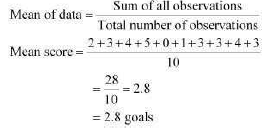Arranging the number of goals in ascending order, 0, 1, 2, 3, 3, 3, 3, 4, 4, 5
The number of observations is 10, which is an even number. Therefore, median score will be the mean of  10/2 i.e., 5th and  10/2+1 ascending or descending order.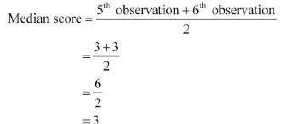i.e., 6 th observation while arranged in Mode of data is the observation with the maximum frequency in data.
Therefore, the mode score of data is 3 as it has the maximum frequency as 4 in the data.

Question : The following observations have been arranged in ascending order. If the median of the data is 63, find the value of x.
29, 32, 48, 50, x, x + 2, 72, 78, 84, 95
It can be observed that the total number of observations in the given data is 10 (even number). Therefore, the median of this data will be the mean of  10/2 i.e., 5th and 10/2 +1 i.e., 6 th observation.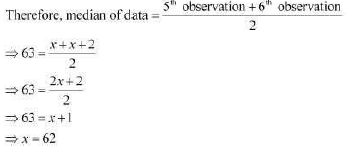STATISTICS

Question : The enrollment of a school during six consecutive years was as follows—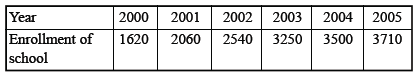(a) Find the mean of the enrollment of the school for this period.

(b) Draw a bar graph of the above data.

(c) What value this data represent ?

(b)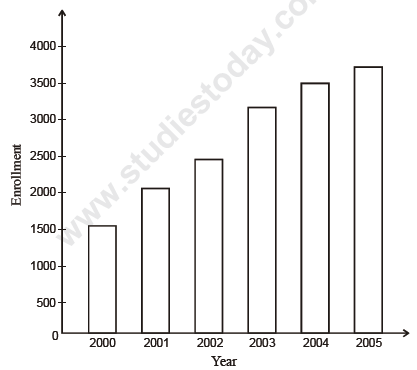(c) Awareness of people towards education

Question : Status of tigers in India in last 100 years is as follows—(a) Make bar graph of above data.

(b) What idea promotes here ?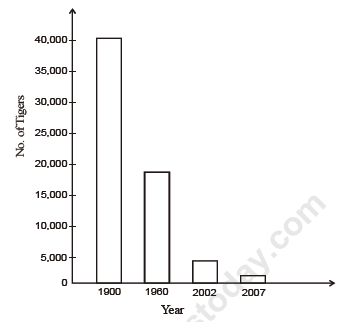Question : The following table shows the interest paid by India (in thousand crore rupees) on external debts during the period 1998-99 to 2002-03.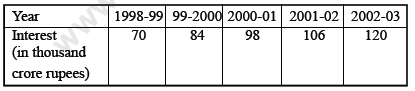(a) Draw histogram of the above data.

(b) What conclusion will derive from above data ?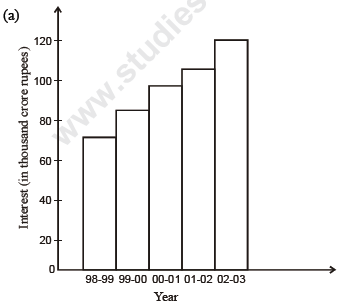(b) We should try to be more self dependent.

Question : The following bar graph represents the budget allocation by Govt. of NCT of Delhi under Ladli scheme.This scheme started in January 2008.

(a) In which year the budget was minimum ?

(b) In which year the budget was maximum ?

(c) What values are depicted from the above data ?
Answer : (a) The budget was minimum in 2007-08.

(b) The budget was maximum in 2010-11.

(c) (i) Save the girl child.

(ii) Improving school enrollment.

(iii) Reducing insecurity among the parents having only daughter.

Question : 25 plants each were planted in 25 schools during Van Mahotsava.
16        20        23      10           6
10        12         7         9          15
15        22        11       13         21
17        9           4        10         12
20        15        18       18         22

(a) Prepare a frequency distribution table of the above data using class interval 0-5 etc.

(b) What values are represented through this activity.
Answer : (a) Class Interval                   No. of plants
0-5                                    1
5-10                                  4
10-15                                7
15-20                                7
20-25                                6

(b) Conservation of earth by tree plantation.

Question : A group of 10 students visited to a blind school. They donated Rs. 100, 120, 125, 50, 75, 100, 150, 100, 75, 85.

(a) Find mean, mode, median of this data.

(b) What impression will you receive through this ?
Answer : (a) By arranging the data in ascending order, we get

50, 75, 75, 85, 100, 100, 120, 125, 150
Mean =980/10 = 80

Mode = 100
Median =( 5th + 6th/2)  term= 100+100/2=200/2=100

(b) Habit of giving donation for social cause

Question : The following data represents the power consumption of Delhi from 2001 to 2010.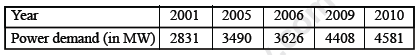(a) Draw bar graph of above data.

(b) What represents through the above data.(b) Save the electricity

Question : The following data represents the cases of deptheria and death due to deptheria—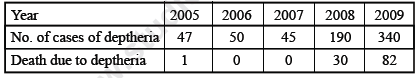(a) In which year, the cases of deptheria were minimum ?

(b) In which year, the deptheria death occurs ?

(c) What value we draw from the above data ?
Answer : (a) In 2007, the cases of deptheria were minimum.

(b) In 2006 and 2007, no death due to deptheria occur.

(c) The vaccination programme should implemented to reduce cases as well as deaths due to deptheria.

(d) Community services

Question : The following data represents the cases of whooping cough in India during the following years—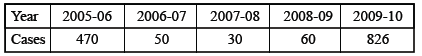(a) Draw frequency polygon curve of the above data.

(b) What value represents this data ?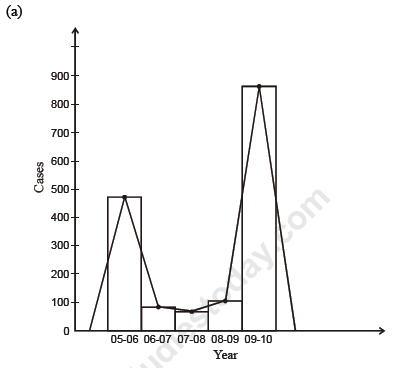(b) Community services

Question : 10 people were asked about the time they spent in a week in doing social work in their community. They said 10, 7, 13, 10, 20, 15, 10, 14, 12, 16 hours resp. Find the mean, mode, median time in a week devoted by them for social work. What value we draw from the above data ?
Answer : (a) Arranging in ascending order
7, 10, 10, 10, 12, 13, 14, 15, 16, 20
Mean =   7+ 10 +10 +10+ 12+ 13+ 14 +15+ 16 +20/10  = 127/10 = 12.7

Mode = 10

Median =5th+ 6th/2 term = 12 +13/2= 25 /2=12.5

(b) Social service
 CBSE Class 9 Mathematics Circles VBQs
 CBSE Class 9 Mathematics Construction VBQs
 CBSE Class 9 Mathematics Surface Areas And Volume VBQs
 CBSE Class 9 Mathematics Statistics VBQs
 CBSE Class 9 Mathematics Probability VBQs
 CBSE Class 9 Mathematics Linear Equations VBQs
 CBSE Class 9 Mathematics Quadrilaterals VBQs
 CBSE Class 9 Mathematics Areas Of Parallelograms And Triangles VBQs
 CBSE Class 9 Mathematics Value Based Questions

Tags